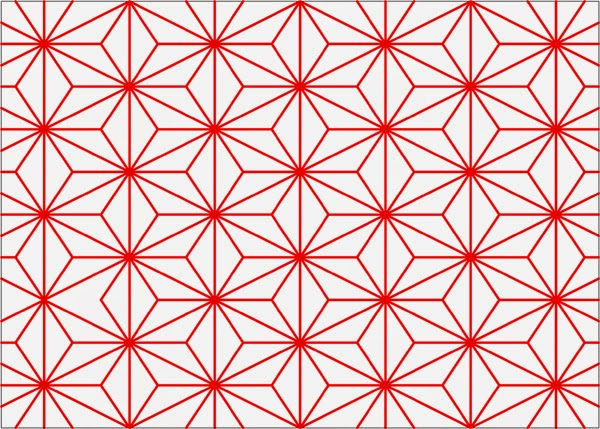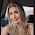##There are many ways to draw the Asa-No-pattern. The following technique is based on tesselation and suggest the same steps as in sashiko embroidery.

Instructions
1. Draw a grid of horizontal and vertical lines at equal intervals (a grid of squares).

2. Draw an oblique line, starting from an intersection point around the middle of the bottom edge and moving two squares right and one square top.

3. Draw all the oblique lines from bottom left to top right. The distance between these lines is 4 squares in the horizontal axis and 2 squares in the vertical axis.

4. Follow the same steps and draw the symmetrical, oblique lines from bottom right to top left.

5. Highlight, with a red line for example, the vertical lines, that connect the intersection points (a,b,c etc) between the oblique lines.

6. Highlight the oblique lines in both directions, creating a grid of triangles.

7. Draw an oblique line to connect diagonally the centers of the polygons a,b,c, ect.

8. Draw all the oblique lines as above.

9. Draw the oblique lines in the opposite direction

10. Highlight the vertical diamonds, from interection point to intersection point, as shown below.

11. Highlight all the vertical diamonds.

12. Add the middle, horizontal lines, inside each star, connecting the points from (a) to (b).

13. Delete the black lines of the grid. The pattern is ready.

Note: In Asa-no-ha tilling, the repeat pattern is a six-pointed star

References:#### 1 comment:

1.thank you so so much! <3